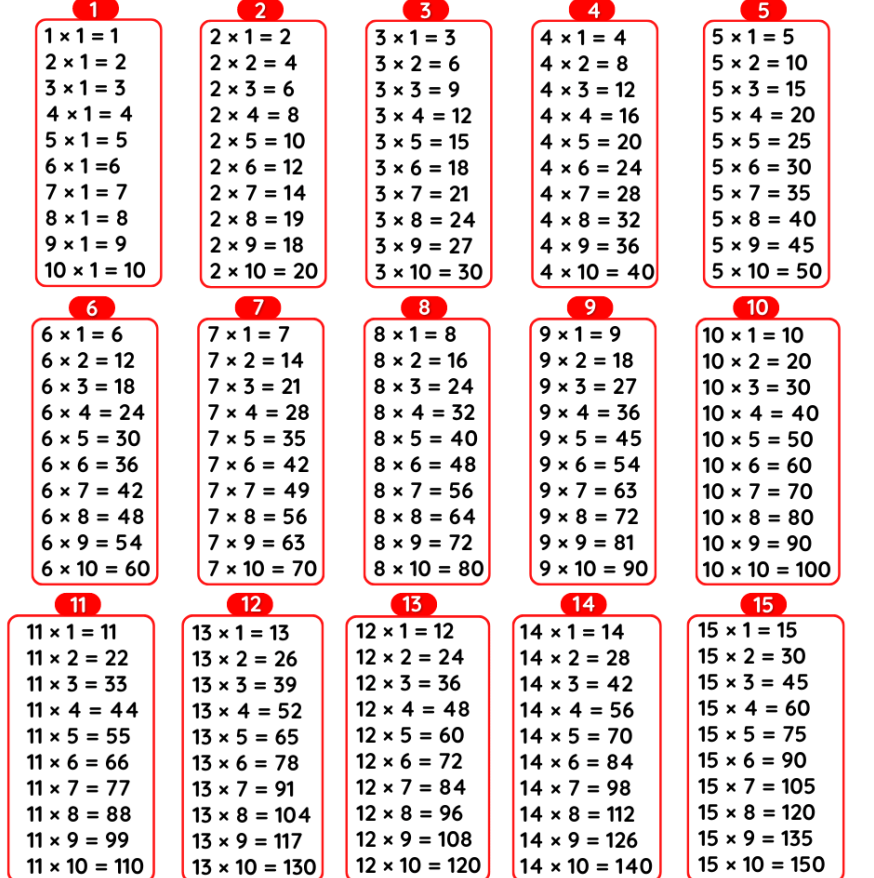# Tables 2 to 15, 2 se 15 tak table, Maths 2 to 15 tables Multiplication Chart

## Tables 2 to 15

Maths Multiplication Tables 2 to 15, Tables 2 to 15, 2 se 15 tak Table, 2 se lekar 15 tak Table, 2 to 15 Table Chart

### Tables 2 to 5

 Table of 2 Table of 3 Table of 4 Table of 5 2 ×‌ 1 = 2 3 × ‌1 = 3 4 × ‌1 = 4 5 × ‌1 = 5 2 ×‌ 2 = 4 3 × ‌2 = 6 4 × ‌2 = 8 5 × ‌2 = 10 2 × ‌3 = 6 3 × ‌3 = 9 4 × ‌3 = 12 5 × ‌3 = 15 2 × ‌4 = 8 3 × ‌4 = 12 4 × ‌4 = 16 5 × ‌4 = 20 2 × ‌5 = 10 3 × ‌5 = 15 4 × ‌5 = 20 5 × ‌5 = 25 2 × ‌6 = 12 3 × ‌6 = 18 4 × ‌6 = 24 5 × ‌6 = 30 2 × ‌7 = 14 3 × ‌7 = 21 4 × ‌7 = 28 5 × ‌7 = 35 2 × ‌8 = 16 3 × ‌8 = 24 4 × ‌8 = 32 5 × 8 = 40 2 × ‌9 = 18 3 × ‌9 = 27 4 × ‌9 = 36 5 × 9 = 45 2 × ‌10 = 20 3 × ‌10 = 30 4 × ‌10 = 40 5 × 10 = 50

### Tables 6 to 10

 Table of 6 Table of 7 Table of 8 Table of 9 Table of 10 6 × 1 = 6 7 × 1 = 7 8 × 1 = 8 9 × 1 = 9 10 × 1 = 10 6 × 2 = 12 7 × 2 = 14 8 × 2 = 16 9 × 2 = 18 10 × 2 = 20 6 × 3 = 18 7 × 3 = 21 8 × 3 = 24 9 × 3 = 27 10 × 3 = 30 6 × 4 = 24 7 × 4 = 28 8 × 4 = 32 9 × 4 = 36 10 × 4 = 40 6 × 5 = 30 7 × 5 = 35 8 × 5 = 40 9 × 5 = 45 10 × 5 = 50 6 × 6 = 36 7 × 6 = 42 8 × 6 = 48 9 × 6 = 54 10 × 6 = 60 6 × 7 = 42 7 × 7 = 49 8 × 7 = 56 9 × 7 = 63 10 × 7 = 70 6 × 8 = 48 7 × 8 = 56 8 × 8 = 64 9 × 8 = 72 10 × 8 = 80 6 × 9 = 54 7 × 9 = 63 8 × 9 = 72 9 × 9 = 81 10 × 9 = 90 6 × 10 = 60 7 × 10 = 70 8 × 10 = 80 9 × 10 = 90 10 × 10 = 100

### Tables 11 to 15

 Table of 11 Table of 12 Table of 13 Table of 14 Table of 15 11 ×‌‌ 1 = 11 12 ×‌ 1 = 12 13 ×‌ 1 = 13 14 ×‌ 1 = 14 15 ×‌ 1 = 15 11 ×‌‌ 2 = 22 12 ×‌ 2 = 24 13 ×‌ 2 = 26 14 ×‌ 2 = 28 15 ×‌ 2 = 30 11 ×‌‌ 3 = 33 12 ×‌ 3 = 36 13 ×‌ 3 = 39 14 ×‌ 3 = 42 15 ×‌ 3 = 45 11 ×‌ 4 = 44 12 ×‌ 4 = 48 13 ×‌ 4 = 52 14 ×‌ 4 = 56 15 ×‌ 4 = 60 11 ×‌ 5 = 55 12 ×‌ 5 = 60 13 ×‌ 5 = 65 14 ×‌ 5 = 70 15 ×‌ 5 = 75 11 ×‌ 6 = 66 12 ×‌ 6 = 72 13 ×‌ 6 = 78 14 ×‌ 6 = 84 15 ×‌ 6 = 90 11 ×‌ 7 = 77 12 ×‌ 7 = 84 13 ×‌ 7 = 91 14 ×‌ 7 = 98 15 ×‌ 7 = 105 11 ×‌ 8 = 88 12 ×‌ 8 = 96 13 ×‌ 8 = 104 14 ×‌ 8 = 112 15 ×‌ 8 = 120 11 ×‌ 9 = 99 12 ×‌ 9 = 108 13 ×‌ 9 = 117 14 ×‌ 9 = 126 15 ×‌ 9 = 135 11 ×‌ 10 = 110 12 ×‌ 10 = 120 13 ×‌ 10 = 130 14 ×‌ 10 = 140 15 ×‌ 10 = 150

### 2 se 15 tak Table, 2 se lekar 15 tak Table### Multiplication Tables 2 to 15### 2 to 15 Table chart

#### Tables 2 to 10#### Tables 11 to 20